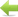11,668 PagesBack to the list of advances

Mathematics is an Ancient Times technology.

## Civilopedia Entry

Rudimentary arithmetic was purely quantitative, used to keep track of quantities and measurements in trade. Building on this fundamental base, ancient civilizations began to formulate more complex mathematical concepts. As early as 1800 BC, the Egyptians had developed a system for working with fractional numbers and simple algebraic problems. They also formulated geometric formulae for finding area and volume, and came up with a constant for determining the area of a circle that was very close to the value of pi. By about the 5th century BC, the mathematicians of Greece had greatly contributed to both geometry and algebra. Around the same time, advanced mathematics was applied to other sciences and fields of study such as astronomy and mechanics. One of the earliest applications of mathematical principles was in the construction of complex weaponry such as the catapult.